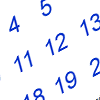Start with the set of the twenty-one numbers 0 – 20.

Can you arrange these numbers into 7 subsets, each of three numbers, so that the totals of the three numbers in each subset make seven consecutive numbers?

For example, one subset adding up to 27 might be {0, 13, 14}. Another adding up to 28 might be {1, 12, 15}. Find three other numbers that add up to 29 ?  These sets are the kind of thing that you need as 27, 28 and 29 are consecutive numbers. Remember that consecutive numbers are numbers which follow each other when you are counting, for example, {24, 25, 26} or {39, 40, 41}.

Hint: Add up all the numbers from 0 to 20. Can you use this sum to decide how big the seven consecutive numbers should be.

There are thousands of number patters that give solutions. What further number patterns can you find? What do you notice about the patterns?

### 9 Responses to Seven Consecutive Numbers

1.Mabhamba Mabhamba says:

I found the solution for the problem by trial and error.
I first added 20+0+7= 27 after many trials but i made sure that i add small numbers with big number.
I altemately found that if i keep on decreasing by 1 to bi numbers and increase by 1 to small numbers that would make the same total. Increasing by 1 to other number will make the total to be 1 greater than previous one.
Therefore:
20+0+7=27
19+1+8=28
18+2+9=29
17+3+10=30
16+4+11=31
15+5+12=32
14+6+13=33

Thats how I got.

2.toni says:

Well done Mabhamba, you have found a beautiful pattern and one of the many thousands of solutions to this problem.

From now on nobody else should put their solutions here.

Give this problem to your learners and use this discussion to share ideas about teaching.

Don’t give your learners too much ‘guidance’. Let them discover the patterns for themselves and eventually discover systematic ways of finding and recording solutions.

Because there are so many solutions this problem can last for many weeks. Give a lot of praise and encouragement to each learner who finds a solution that nobody has found before. Don’t do any ‘spoon feeding’.

3.Buyi Taho says:

I will be using this problem with my grade 11. I will share my experience when finished.

4.Change Qazisa says:

This is a good and encouraging activity ,very challenging with a variety of solutions ,definately i will give it to my Grade 9 learners to see their solutions on this activity.

5.Nelly Malamlela says:

i got many patterns from my learners as solutions to this problem. learners can suprise yu sometimes. the type of responces i got; i didnt even thought about them before.

6.Ntyatyi Ndarala says:

With my learners they had a problem with intepreting the problem,that is of knowing the meaning of consecutive numbers but after that it was fine we.’ve also used the numbers in building the patterns

7.Buyi Taho says:

The learners struggled to get the consecutive numbers from the sequence. They manage to restrict themselves that a number must be used once. Interesting enough most of them their solutions were not the same. The was a group that proved that the sum of the sequence formed is equal to the numbers given. They were excited with this activity.

8.Nomandla Mbobo says:

I think i enjoyed the two solutions from Mabhamba and Ntholeng,they can be understood by my learners who always take a long time to follow the instructions.The solutions are clear and the level is for the gradegrades that I am teaching.

9.Lithas Sokweba says:

It is interesting to here other teachers sharing their problems